Next: 11. Theory Ensembles Up: 2. User's Guide Previous: 9. Theory Interpretations

Subsections

# 10. Quasi-Constructors

The purpose of this chapter is fourfold:

1.
To explain why quasi-constructors are desirable.
2.
To describe how they are implemented.
3.
To show how they are created and reasoned with.
4.
To point out the pitfalls associated with their use.

# 10.1 Motivation

The constructors of LUTINS are fixed, but you can define quasi-constructors which effectively serve as additional constructors. Quasi-constructors are desirable for several reasons:

1.
Once a quasi-constructor is defined, it is available in every theory whose language contains the quasi-constructor's home language. That is, a quasi-constructor is a kind of global constant that can be freely used across a large class of theories. (A constructor, as a logical constant, is available in every theory.)

2.
Quasi-constructors are polymorphic in the sense that they can be applied to expressions of several different types. (Several of the constructors, such as = and if, are also polymorphic in this sense.)

3.
The definition of a translation (see Chapter 9) is not directly dependent on the definition of any quasi-constructor. Consequently, translations can be applied to expressions involving quasi-constructors that were defined after the translation itself was defined.

4.
Quasi-constructors can be defined without modifying the IMPS deductive machinery. (Note that the deductive machinery of a ordinary constructor is hard-wired'' into IMPS.)

5.
Quasi-constructors can be used to represent operators in nonclassical logics and operators on generic objects likes sets and sequences.

# 10.2 Implementation

Quasi-constructors are implemented as macro/abbreviations.'' For example, the quasi-constructor quasi-equals10.1 is defined by the following biconditional: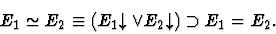Hence, two expressions are quasi-equal if, and only if, they are either both undefined or both defined with the same value.

When the preparsed string representation of an expression (preparsed string'' for short) of the form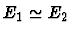is parsed,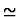is treated as a macro with the list of arguments E1,E2: an internal representation of the expression (internal expression'') is built from the preparsed string as if it had the form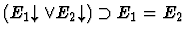. When an internal expression of the formis printed,is used as an abbreviation: the printed string representation of the expression (printed string'') is generated from the internal expression as if the latter had the form. In other words, in string representation of the expression looks like, an operator applied to a list of two arguments, but it is actually represented internally as.

Abstractly, a quasi-constructor definition consists of three components: a name, a list of schema variables, and a schema. In our example above, quasi-equals is the name, E1,E2 is the list of schema variables, and the right-hand side of the biconditional above is the schema. A quasi-constructor is defined by a user with the def-quasi-constructor form. The list of schema variables and the schema are represented together by a lambda-expression which is specified by a string and a name of a language or theory. The variables of the lambda-expression represent the list of schema variables, and the body of the lambda-expression represents the schema.

For instance, quasi-equals could be defined by:

   (def-quasi-constructor QEQUALS
"lambda(e1,e2:ind, #(e1) or #(e2) implies e1 = e2)"
(language the-kernel-theory))

The name of the quasi-constructor is qequals, and the lambda-expression is the expression specified by the string in the-kernel-theory. After this form has been evaluated, an expression of the form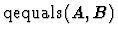is well-formed in any language containing the language of the-kernel-theory, provided A and B are well-formed expressions of the same type of kind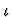. Separate from the definition, special syntax may be defined for parsing and printing qequals (e.g., as the infix symbol).

The quasi-constructor quasi-equals is not actually defined using a def-quasi-constructor form in IMPS. It is one of a small number of system quasi-constructors'' which have been directly defined by the IMPS implementors. A system quasi-constructor cannot be defined using the def-quasi-constructor form because the schema of the quasi-constructor cannot be fully represented as a LUTINS lambda-expression. This is usually because one or more variables of the schema variables ranges over function expressions of variable arity or over expressions of both kindand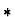. For example,is not well-defined if A and B are of kind, but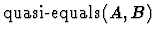is well-defined as long as A and B have the same type (regardless of its kind). The major system quasi-constructors are listed in Table 10.1.

Table 10.1: System Quasi-Constructors
 Quasi-constructor Schema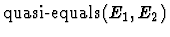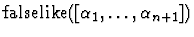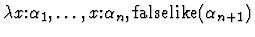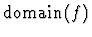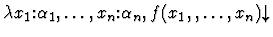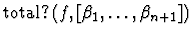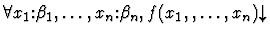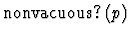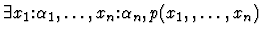Notes:
• E1 and E2 must have the same type.
•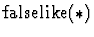represents the same internal expression as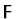.
• The sort of f is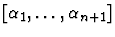.
•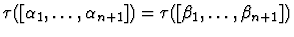.
• The sort of p is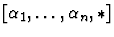# 10.3 Reasoning with Quasi-Constructors

There are two modes for reasoning with a quasi-constructor Q. In the enabled mode the internal structure of Q is ignored. For example, in this mode, when an expression Q(E1,..,En) is simplified, only the parts of the internal expression corresponding to the arguments E1,..,En are simplified, and the part corresponding to Q is skipped.'' Similarly, when a macete is applied to an expression Q(E1,..,En), subexpressions located in the part of the internal expression corresponding to Q are ignored. In this mode, quasi-constructors are intended to behave as if they were ordinary constructors.

In the disabled mode, the internal structure of Q has no special status. That is, deduction is performed on internal expressions as if Q was never defined. (However, in this mode expressions involving Q will still be printed using Q as an abbreviation.) The mode is activated by disabling Q,'' and it inactivated by enabling Q.'' The disabled mode is used for proving basic properties about Q. Once a good set of basic properties are proven and formalized as theorems, there is usually little need for the disabled mode. Most quasi-constructors are intended to be employed in multiple theories. Hence, you will usually want to install a theorem about a quasi-constructor with the usage transportable-macete.

For a few of the interactive proof commands, there is a dual command with the word insistent'' put somewhere into the command's name. For example, the dual of simplify is the simplify-insistently. Calling the dual of a command C is equivalent to disabling all quasi-constructors and then calling Citself. In other words, the dual commands behave as if there were no quasi-constructors at all. These insistent'' commands are intended to be used sparingly because they can disturb the internal structure corresponding to a quasi-constructor, creating a new internal expression that can no longer be abbreviated by the quasi-constructor. In visual terms, the quasi-constructor explodes.''

One of the advantages of working in a logic like LUTINS, with a rich structure of functions, is that generic objects like sets and sequences can be represented directly in the logic as certain kinds of functions. For instance, sets are represented in IMPS as indicators, which are similar to characteristic functions, except that x is a member'' of an indicator f iff f(x) is defined. Operators on indicators and other functions representing generic objects are formalized in IMPS as quasi-constructors, and theorems about these operators are proved in generic theories'' that contain neither constants nor axioms (except for possibly the axioms of the theory h-o-real-arithmetic). Consequently, reasoning is performed in generic theories using only the purely logical apparatus of LUTINS (and possibly h-o-real-arithmetic). Moreover, theorems about generic objects are easy to apply in other theories since the operators, as quasi-constructors, are polymorphic and since the theory interpretations involved usually have no obligations to check.

# 10.4 Hints and Cautions

1.
Reasoning with quasi-constructors can be tricky because the internal representation of the quasi-constructor--the structure it abbreviates--is hidden from view. The view-expr form (see Chapter 17) can be used to print an expression without quasi-constructor abbreviations. For example, when
   (view-expr
"1/0==?zz"
(language-name h-o-real-arithmetic)
no-quasi-constructors)

is evaluated, the expression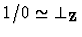is printed as
   (#(1/0) or #(?zz)) implies 1/0=?zz.


2.
It is often easy to confuse quasi-constructors with constants. For instance, a common mistake is to try to unfold a quasi-constructor. To help you avoid such confusion, curly brackets can be used to surround the operands of a quasi-constructor instead of parentheses. That is, a string q{e1,...,en}, where q is a quasi-constructor, is parsed exactly like q(e1,...,en). In addition, the internal expression is always printed like q{e1,...,en}, unless you have defined a special print syntax for q.

3.
It should always be remembered that the internal representation of an expression containing quasi-constructors might be much larger than what one might expect from the printed representation of the expression, especially if the quasi-constructors are defined in terms of other quasi-constructors. Consequently, the processing speed of IMPS on expressions containing quasi-constructors sometimes seems unjustifiably slow.

4.
An expression may sometimes implode'' when parsed. That is, quasi-constructors may appear in the printed string in places where they did not occur in the preparsed string. This happens because the IMPS printing routine will use quasi-constructors as abbreviations wherever possible, regardless of whether these quasi-constructors were used in the preparsed string. Expression implosion can be caused by simplification and macete application.

5.
Due to the implosion phenomenon, the preparsed and printed strings may not parse into the same internal expressions (but the two internal expressions will always be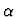-equivalent). For example, consider the preparsed string S1
   with(f:[ind,ind], forall(x:ind, #(f(x))))

It parses into an internal expression E1 which is printed as the string S2
   with(f:[ind,ind],total_q{f,[ind,ind]})

with quasi-constructor abbreviations and is printed as S1 without quasi-constructor abbreviations. However, S2 parses into an internal expression E2 which is printed as S2 with quasi-constructor abbreviations and is printed as the string S3
   with(f:[ind,ind],forall(x_0:ind,#(f(x_0))))

without quasi-constructor abbreviations. That is, the two internal expressions are the same except for the name of the single bound variable.

6.
One of the most devastating forms of quasi-constructor explosion can occur when a nonnormal translation (which maps some atomic sorts to unary predicates) is applied to an expression containing quasi-constructors. The quasi-constructor explosion happens because the binding expressions in the internal representation of a quasi-constructor will be rewritten when the translation is applied if the variables being bound involve a atomic sort which is mapped to a unary predicate.

7.
Often two different internal expressions containing quasi-constructors will be printed in exactly the same way. Usually this is due to the fact that some of the bound variables in the internal representation of some of the quasi-constructors have different names (i.e., the two expressions are-equivalent). In this case, there is no problem; for the most part, IMPS will treat the expressions as if they were identical. However, the two expressions may differ in other ways, e.g., some of the corresponding bound variables may have different sorts. Hence, in rare occasions, it may happen that there are two internal expressions printed the same which are not known by IMPS to be equal (or quasi-equal). This situation can be very hard to deal with. It is often best to backup and try a different path that will avoid this confusing situation.

8.
A quasi-constructor can be disabled, and then later enabled, in the midst of a deduction using the appropriate proof commands. However, disabling a quasi-constructor in the midst of a proof can cause severe confusion if one forgets to later enable it. As a general rule, you should always try to find ways to avoid disabling quasi-constructors.Next: 11. Theory Ensembles Up: 2. User's Guide Previous: 9. Theory Interpretations